Скачать презентацию Engineering Economic Analysis Chapter 7 Rate of Return

55a528dc2fb02e052589c2d64e16cfb8.ppt

• Количество слайдов: 39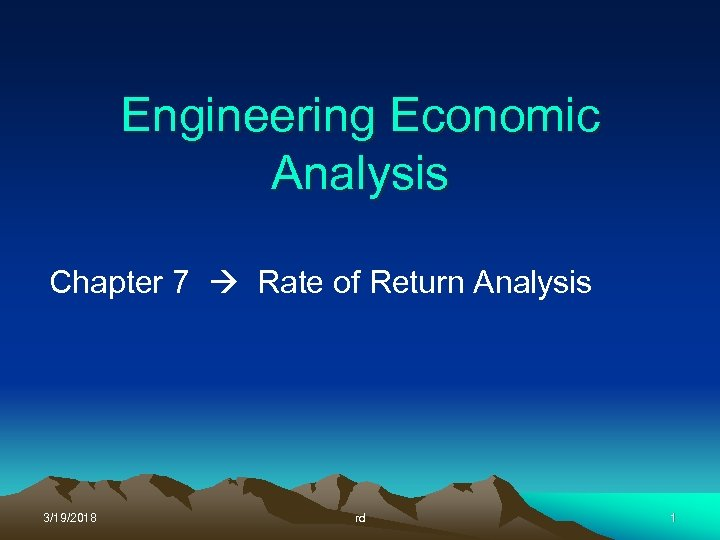Engineering Economic Analysis Chapter 7 Rate of Return Analysis 3/19/2018 rd 1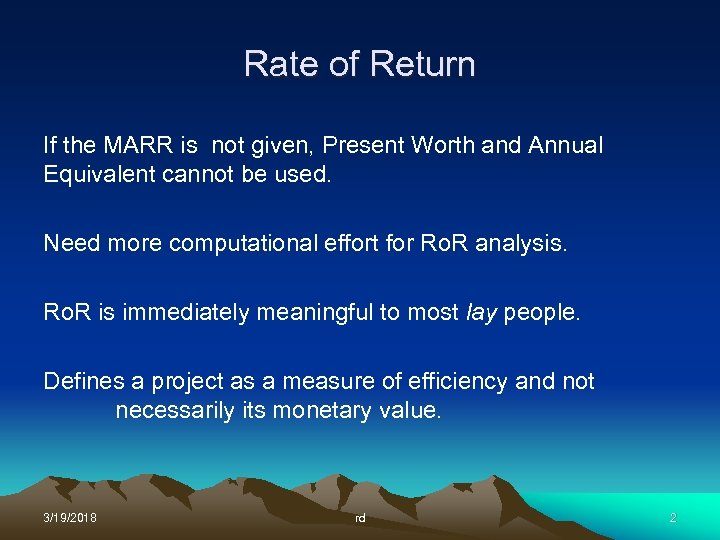Rate of Return If the MARR is not given, Present Worth and Annual Equivalent cannot be used. Need more computational effort for Ro. R analysis. Ro. R is immediately meaningful to most lay people. Defines a project as a measure of efficiency and not necessarily its monetary value. 3/19/2018 rd 2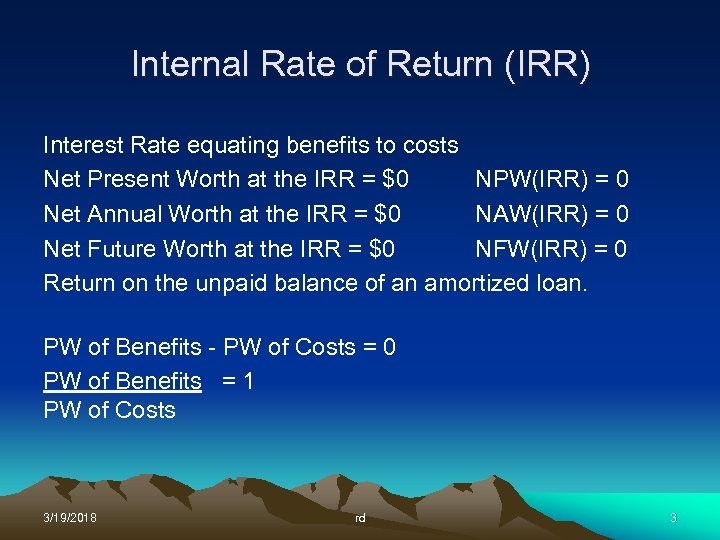Internal Rate of Return (IRR) Interest Rate equating benefits to costs Net Present Worth at the IRR = \$0 NPW(IRR) = 0 Net Annual Worth at the IRR = \$0 NAW(IRR) = 0 Net Future Worth at the IRR = \$0 NFW(IRR) = 0 Return on the unpaid balance of an amortized loan. PW of Benefits - PW of Costs = 0 PW of Benefits = 1 PW of Costs 3/19/2018 rd 3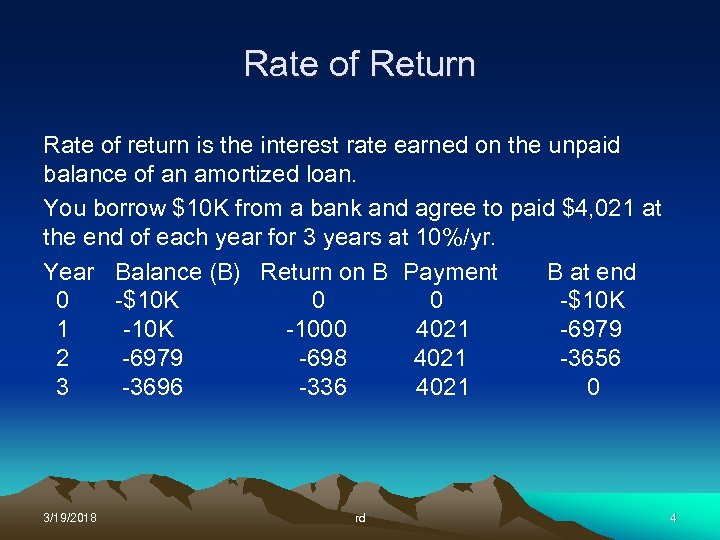Rate of Return Rate of return is the interest rate earned on the unpaid balance of an amortized loan. You borrow \$10 K from a bank and agree to paid \$4, 021 at the end of each year for 3 years at 10%/yr. Year Balance (B) Return on B Payment B at end 0 -\$10 K 0 0 -\$10 K 1 -10 K -1000 4021 -6979 2 -6979 -698 4021 -3656 3 -3696 -336 4021 0 3/19/2018 rd 4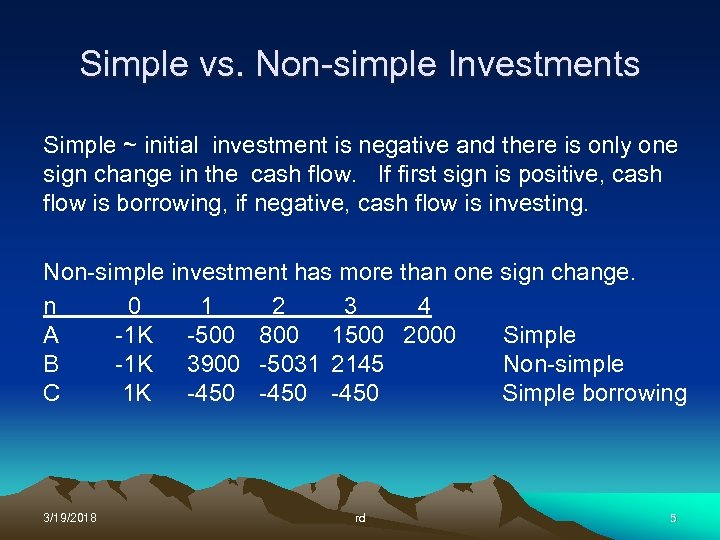Simple vs. Non-simple Investments Simple ~ initial investment is negative and there is only one sign change in the cash flow. If first sign is positive, cash flow is borrowing, if negative, cash flow is investing. Non-simple investment has more than one sign change. n 0 1 2 3 4 A -1 K -500 800 1500 2000 Simple B -1 K 3900 -5031 2145 Non-simple C 1 K -450 Simple borrowing 3/19/2018 rd 5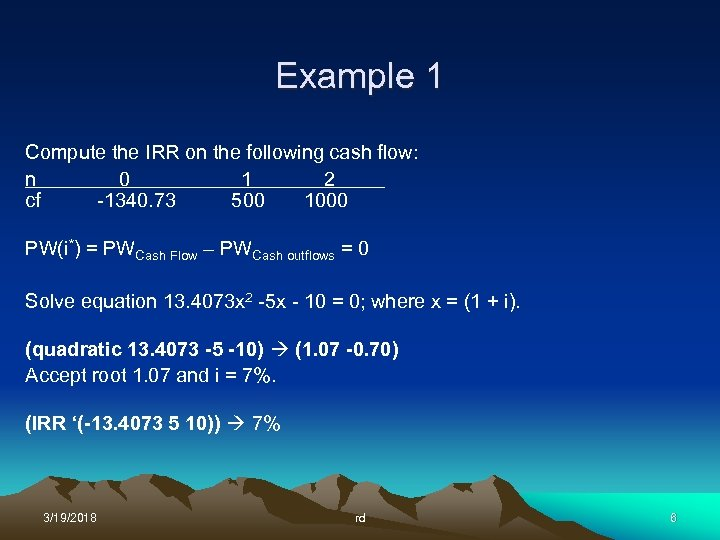Example 1 Compute the IRR on the following cash flow: n 0 1 2 cf -1340. 73 500 1000 PW(i*) = PWCash Flow – PWCash outflows = 0 Solve equation 13. 4073 x 2 -5 x - 10 = 0; where x = (1 + i). (quadratic 13. 4073 -5 -10) (1. 07 -0. 70) Accept root 1. 07 and i = 7%. (IRR ‘(-13. 4073 5 10)) 7% 3/19/2018 rd 6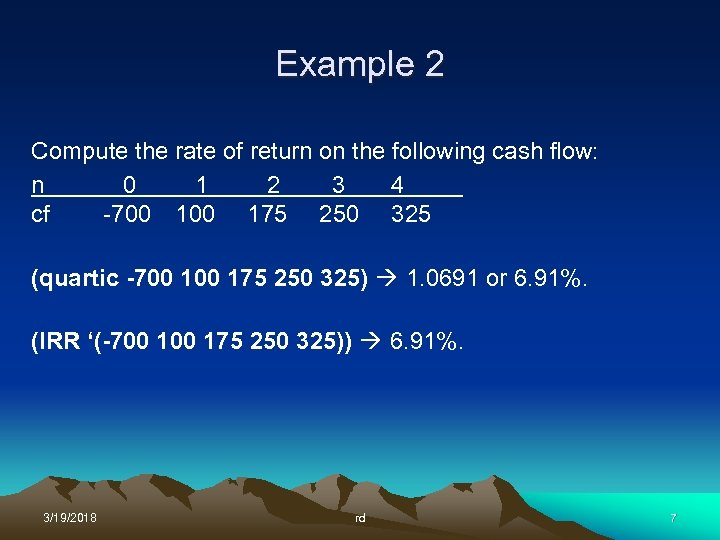Example 2 Compute the rate of return on the following cash flow: n 0 1 2 3 4 cf -700 175 250 325 (quartic -700 175 250 325) 1. 0691 or 6. 91%. (IRR ‘(-700 175 250 325)) 6. 91%. 3/19/2018 rd 7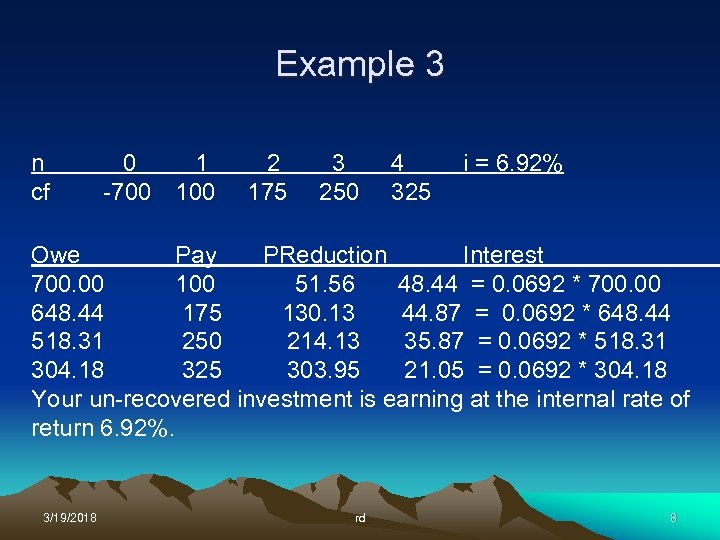Example 3 n cf 0 1 -700 100 2 175 3 250 4 325 i = 6. 92% Owe Pay PReduction Interest 700. 00 100 51. 56 48. 44 = 0. 0692 * 700. 00 648. 44 175 130. 13 44. 87 = 0. 0692 * 648. 44 518. 31 250 214. 13 35. 87 = 0. 0692 * 518. 31 304. 18 325 303. 95 21. 05 = 0. 0692 * 304. 18 Your un-recovered investment is earning at the internal rate of return 6. 92%. 3/19/2018 rd 8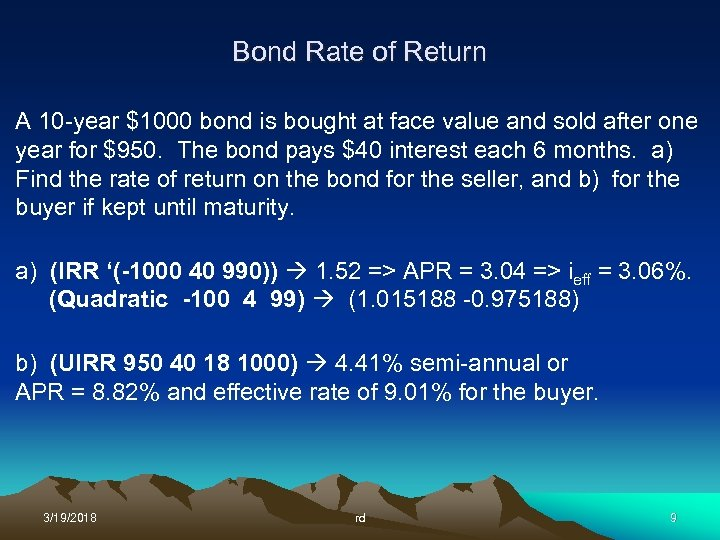Bond Rate of Return A 10 -year \$1000 bond is bought at face value and sold after one year for \$950. The bond pays \$40 interest each 6 months. a) Find the rate of return on the bond for the seller, and b) for the buyer if kept until maturity. a) (IRR ‘(-1000 40 990)) 1. 52 => APR = 3. 04 => ieff = 3. 06%. (Quadratic -100 4 99) (1. 015188 -0. 975188) b) (UIRR 950 40 18 1000) 4. 41% semi-annual or APR = 8. 82% and effective rate of 9. 01% for the buyer. 3/19/2018 rd 9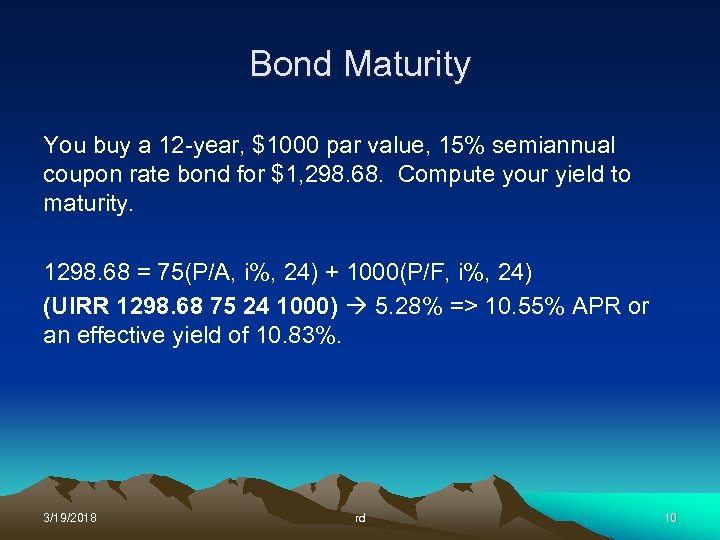Bond Maturity You buy a 12 -year, \$1000 par value, 15% semiannual coupon rate bond for \$1, 298. 68. Compute your yield to maturity. 1298. 68 = 75(P/A, i%, 24) + 1000(P/F, i%, 24) (UIRR 1298. 68 75 24 1000) 5. 28% => 10. 55% APR or an effective yield of 10. 83%. 3/19/2018 rd 10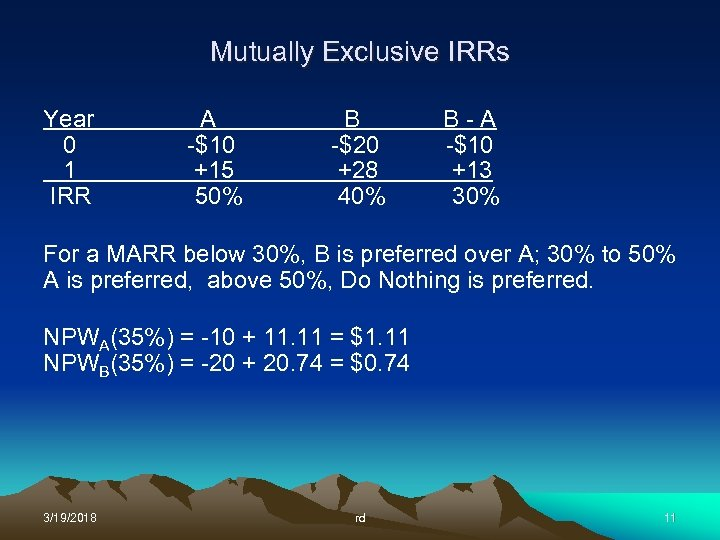Mutually Exclusive IRRs Year 0 1 IRR A -\$10 +15 50% B -\$20 +28 40% B-A -\$10 +13 30% For a MARR below 30%, B is preferred over A; 30% to 50% A is preferred, above 50%, Do Nothing is preferred. NPWA(35%) = -10 + 11. 11 = \$1. 11 NPWB(35%) = -20 + 20. 74 = \$0. 74 3/19/2018 rd 11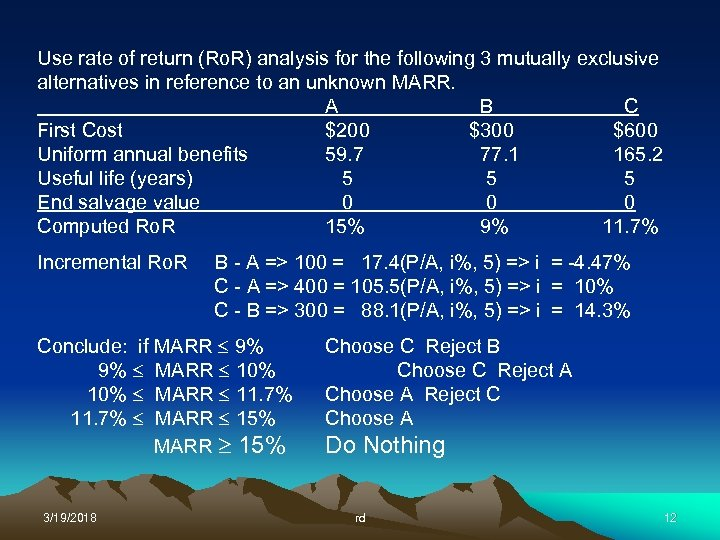Use rate of return (Ro. R) analysis for the following 3 mutually exclusive alternatives in reference to an unknown MARR. A B C First Cost \$200 \$300 \$600 Uniform annual benefits 59. 7 77. 1 165. 2 Useful life (years) 5 5 5 End salvage value 0 0 0 Computed Ro. R 15% 9% 11. 7% Incremental Ro. R B - A => 100 = 17. 4(P/A, i%, 5) => i = -4. 47% C - A => 400 = 105. 5(P/A, i%, 5) => i = 10% C - B => 300 = 88. 1(P/A, i%, 5) => i = 14. 3% Conclude: if MARR 9% 9% MARR 10% MARR 11. 7% MARR 15% 3/19/2018 Choose C Reject B Choose C Reject A Choose A Reject C Choose A Do Nothing rd 12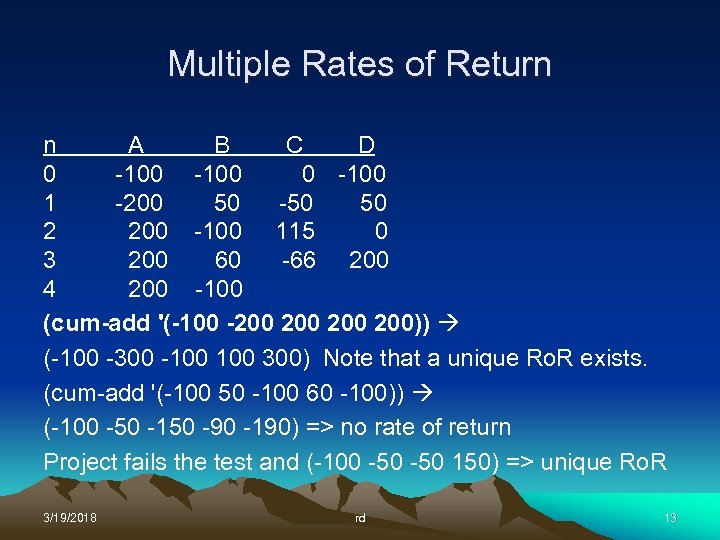Multiple Rates of Return n A B C D 0 -100 1 -200 50 -50 50 2 200 -100 115 0 3 200 60 -66 200 4 200 -100 (cum-add '(-100 -200 200 200)) (-100 -300 -100 300) Note that a unique Ro. R exists. (cum-add '(-100 50 -100 60 -100)) (-100 -50 -150 -90 -190) => no rate of return Project fails the test and (-100 -50 150) => unique Ro. R 3/19/2018 rd 13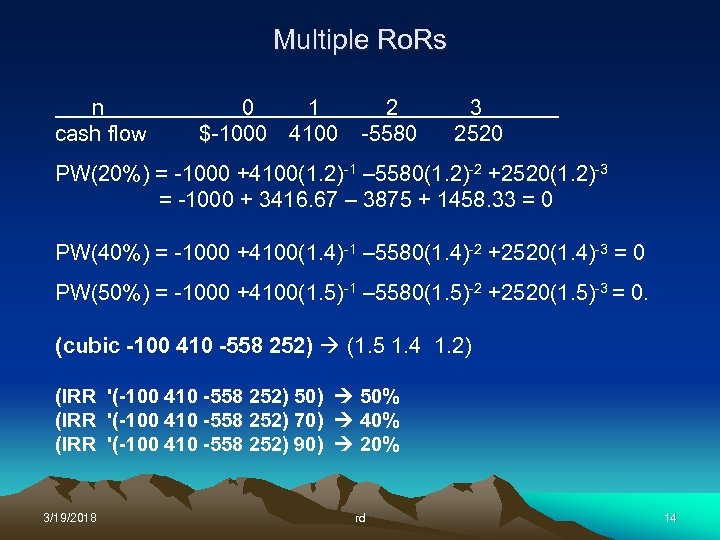Multiple Ro. Rs n cash flow 0 1 \$-1000 4100 2 -5580 3 2520 PW(20%) = -1000 +4100(1. 2)-1 – 5580(1. 2)-2 +2520(1. 2)-3 = -1000 + 3416. 67 – 3875 + 1458. 33 = 0 PW(40%) = -1000 +4100(1. 4)-1 – 5580(1. 4)-2 +2520(1. 4)-3 = 0 PW(50%) = -1000 +4100(1. 5)-1 – 5580(1. 5)-2 +2520(1. 5)-3 = 0. (cubic -100 410 -558 252) (1. 5 1. 4 1. 2) (IRR '(-100 410 -558 252) 50) 50% (IRR '(-100 410 -558 252) 70) 40% (IRR '(-100 410 -558 252) 90) 20% 3/19/2018 rd 14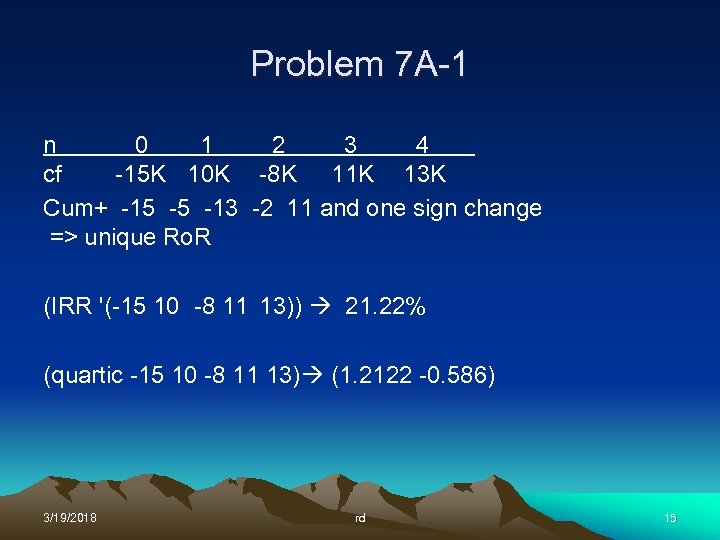Problem 7 A-1 n 0 1 2 3 4 cf -15 K 10 K -8 K 11 K 13 K Cum+ -15 -5 -13 -2 11 and one sign change => unique Ro. R (IRR '(-15 10 -8 11 13)) 21. 22% (quartic -15 10 -8 11 13) (1. 2122 -0. 586) 3/19/2018 rd 15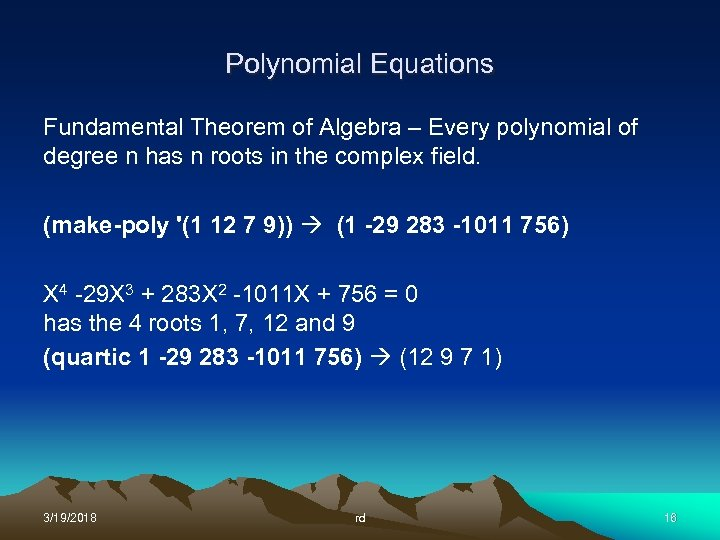Polynomial Equations Fundamental Theorem of Algebra – Every polynomial of degree n has n roots in the complex field. (make-poly '(1 12 7 9)) (1 -29 283 -1011 756) X 4 -29 X 3 + 283 X 2 -1011 X + 756 = 0 has the 4 roots 1, 7, 12 and 9 (quartic 1 -29 283 -1011 756) (12 9 7 1) 3/19/2018 rd 16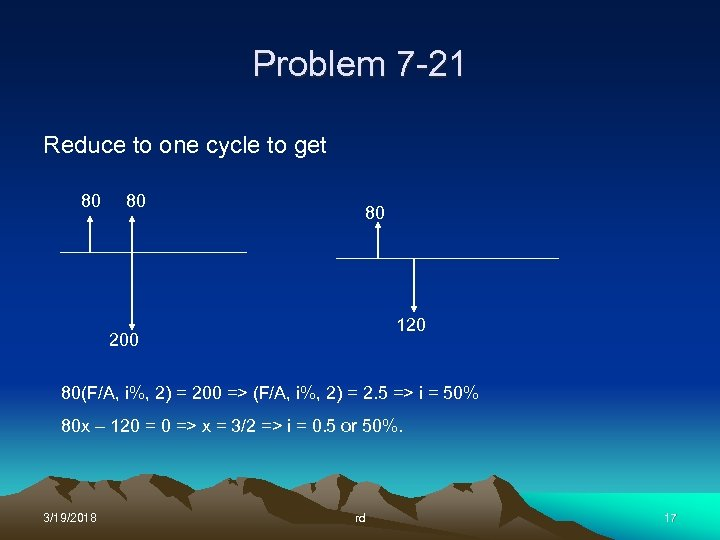Problem 7 -21 Reduce to one cycle to get 80 80 80 120 200 80(F/A, i%, 2) = 200 => (F/A, i%, 2) = 2. 5 => i = 50% 80 x – 120 = 0 => x = 3/2 => i = 0. 5 or 50%. 3/19/2018 rd 17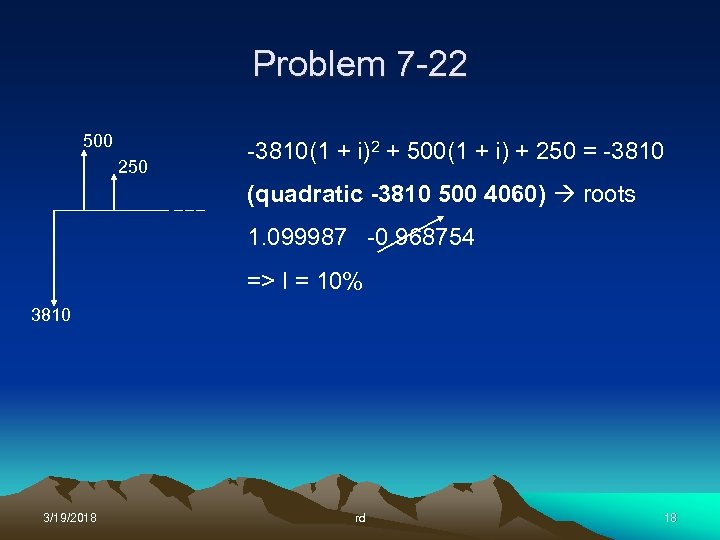Problem 7 -22 500 250 -3810(1 + i)2 + 500(1 + i) + 250 = -3810 (quadratic -3810 500 4060) roots 1. 099987 -0. 968754 => I = 10% 3810 3/19/2018 rd 18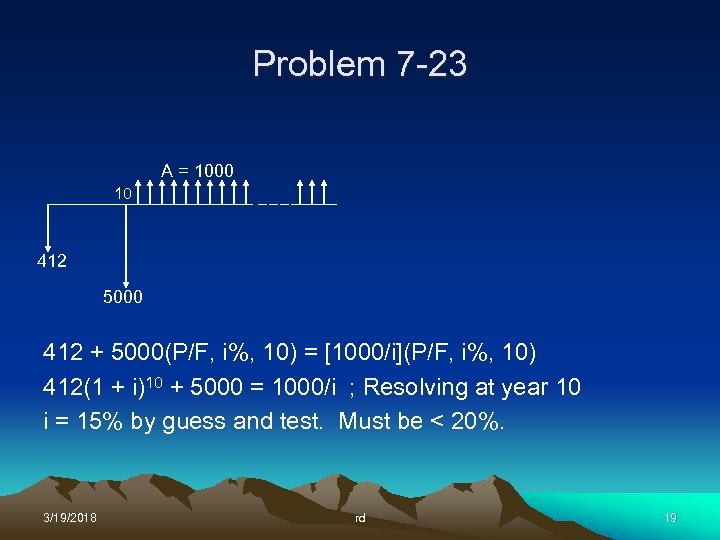Problem 7 -23 A = 1000 10 412 5000 412 + 5000(P/F, i%, 10) = [1000/i](P/F, i%, 10) 412(1 + i)10 + 5000 = 1000/i ; Resolving at year 10 i = 15% by guess and test. Must be < 20%. 3/19/2018 rd 19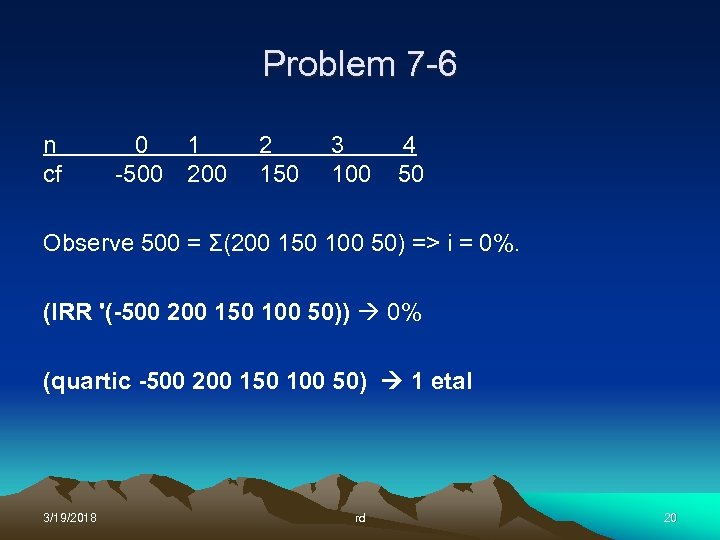Problem 7 -6 n cf 0 1 -500 2 150 3 100 4 50 Observe 500 = Σ(200 150 100 50) => i = 0%. (IRR '(-500 200 150 100 50)) 0% (quartic -500 200 150 100 50) 1 etal 3/19/2018 rd 20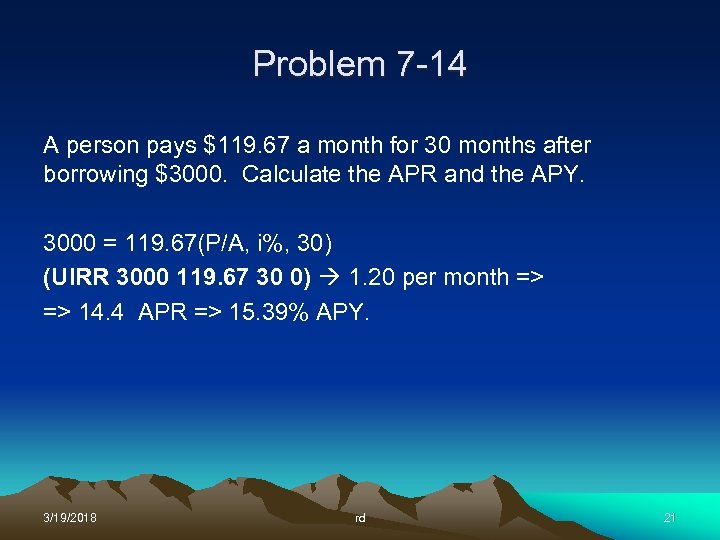Problem 7 -14 A person pays \$119. 67 a month for 30 months after borrowing \$3000. Calculate the APR and the APY. 3000 = 119. 67(P/A, i%, 30) (UIRR 3000 119. 67 30 0) 1. 20 per month => => 14. 4 APR => 15. 39% APY. 3/19/2018 rd 21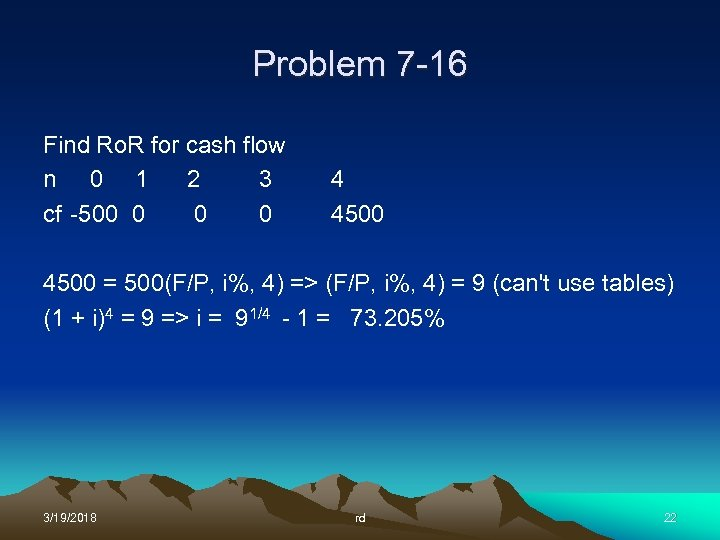Problem 7 -16 Find Ro. R for cash flow n 0 1 2 3 cf -500 0 4 4500 = 500(F/P, i%, 4) => (F/P, i%, 4) = 9 (can't use tables) (1 + i)4 = 9 => i = 91/4 - 1 = 73. 205% 3/19/2018 rd 22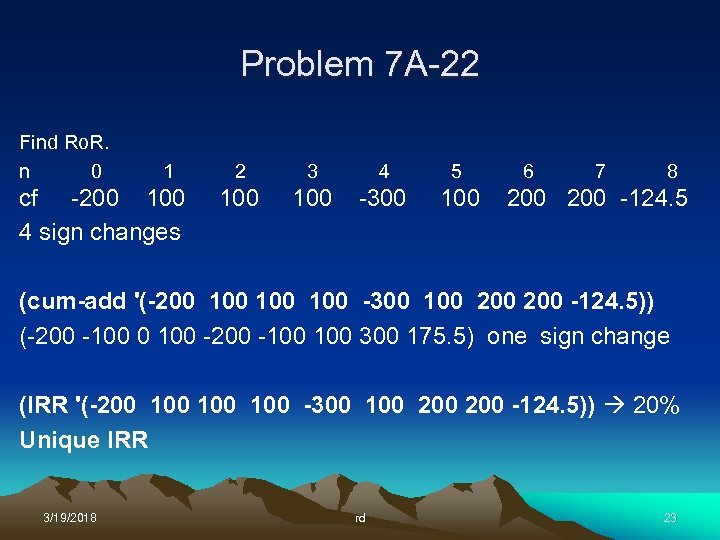Problem 7 A-22 Find Ro. R. n 0 1 cf -200 100 4 sign changes 2 3 4 5 100 -300 100 6 7 8 200 -124. 5 (cum-add '(-200 100 100 -300 100 200 -124. 5)) (-200 -100 0 100 -200 -100 300 175. 5) one sign change (IRR '(-200 100 100 -300 100 200 -124. 5)) 20% Unique IRR 3/19/2018 rd 23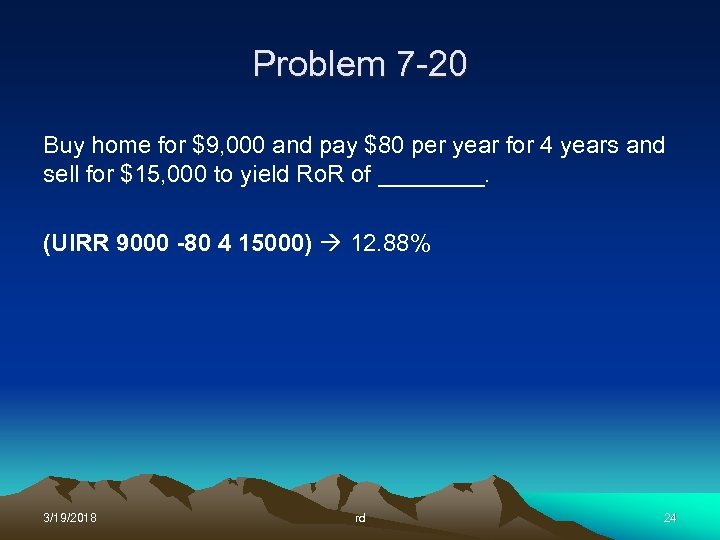Problem 7 -20 Buy home for \$9, 000 and pay \$80 per year for 4 years and sell for \$15, 000 to yield Ro. R of ____. (UIRR 9000 -80 4 15000) 12. 88% 3/19/2018 rd 24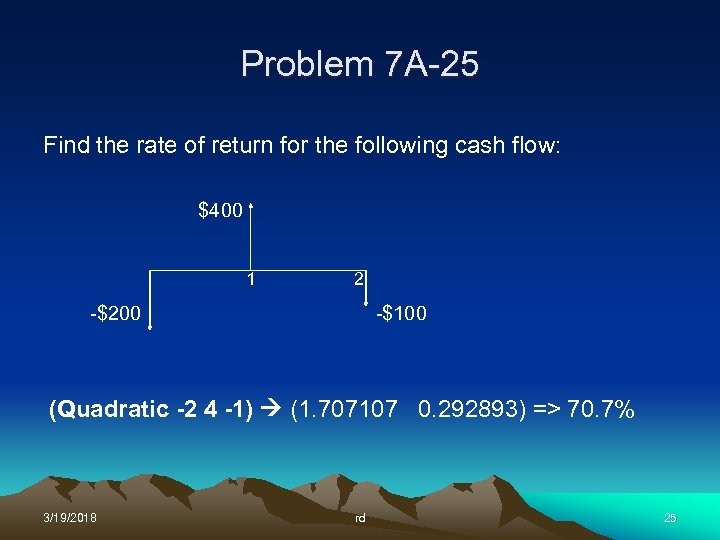Problem 7 A-25 Find the rate of return for the following cash flow: \$400 1 2 -\$200 -\$100 (Quadratic -2 4 -1) (1. 707107 0. 292893) => 70. 7% 3/19/2018 rd 25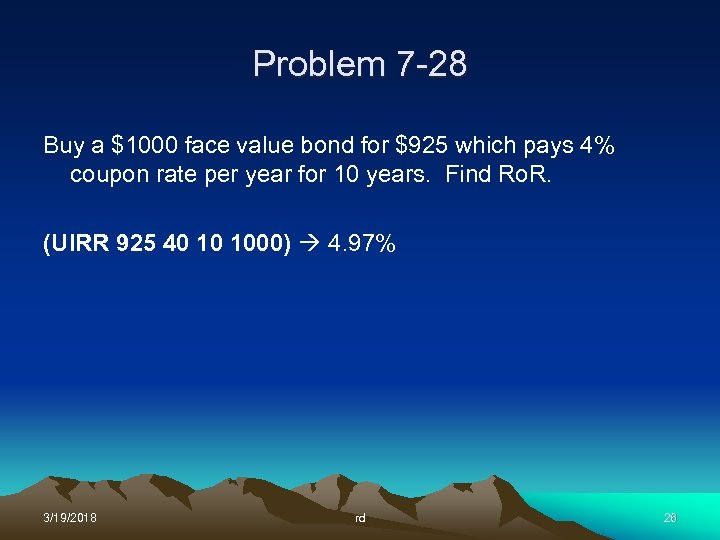Problem 7 -28 Buy a \$1000 face value bond for \$925 which pays 4% coupon rate per year for 10 years. Find Ro. R. (UIRR 925 40 10 1000) 4. 97% 3/19/2018 rd 26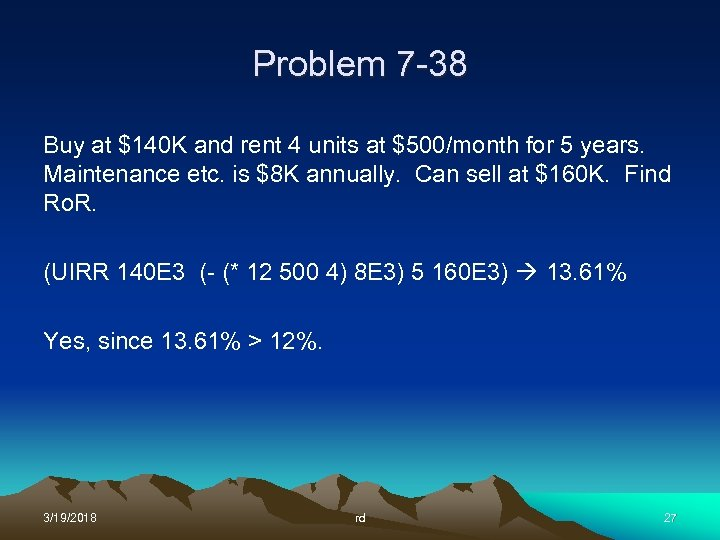Problem 7 -38 Buy at \$140 K and rent 4 units at \$500/month for 5 years. Maintenance etc. is \$8 K annually. Can sell at \$160 K. Find Ro. R. (UIRR 140 E 3 (- (* 12 500 4) 8 E 3) 5 160 E 3) 13. 61% Yes, since 13. 61% > 12%. 3/19/2018 rd 27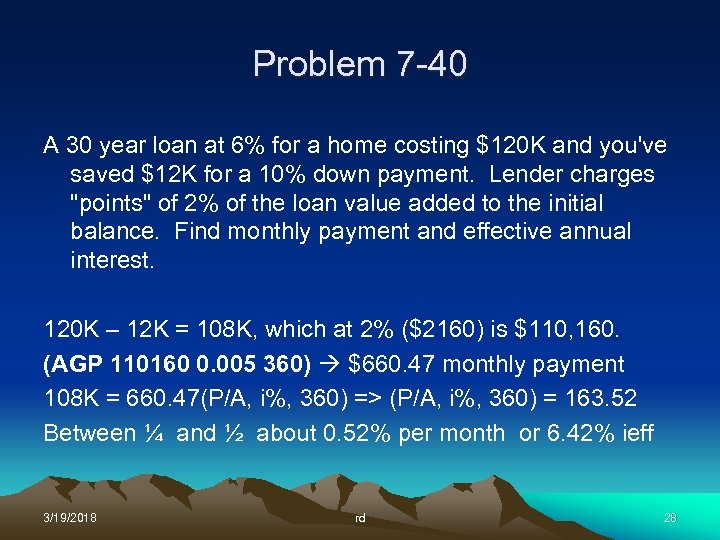Problem 7 -40 A 30 year loan at 6% for a home costing \$120 K and you've saved \$12 K for a 10% down payment. Lender charges "points" of 2% of the loan value added to the initial balance. Find monthly payment and effective annual interest. 120 K – 12 K = 108 K, which at 2% (\$2160) is \$110, 160. (AGP 110160 0. 005 360) \$660. 47 monthly payment 108 K = 660. 47(P/A, i%, 360) => (P/A, i%, 360) = 163. 52 Between ¼ and ½ about 0. 52% per month or 6. 42% ieff 3/19/2018 rd 28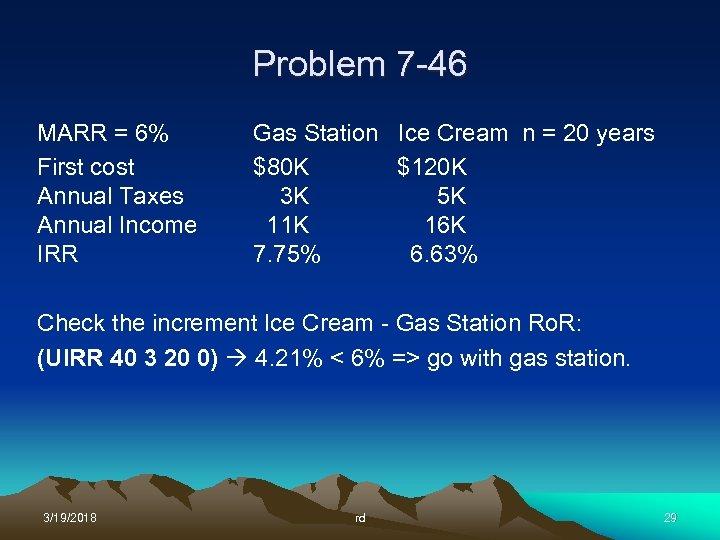Problem 7 -46 MARR = 6% First cost Annual Taxes Annual Income IRR Gas Station Ice Cream n = 20 years \$80 K \$120 K 3 K 5 K 11 K 16 K 7. 75% 6. 63% Check the increment Ice Cream - Gas Station Ro. R: (UIRR 40 3 20 0) 4. 21% < 6% => go with gas station. 3/19/2018 rd 29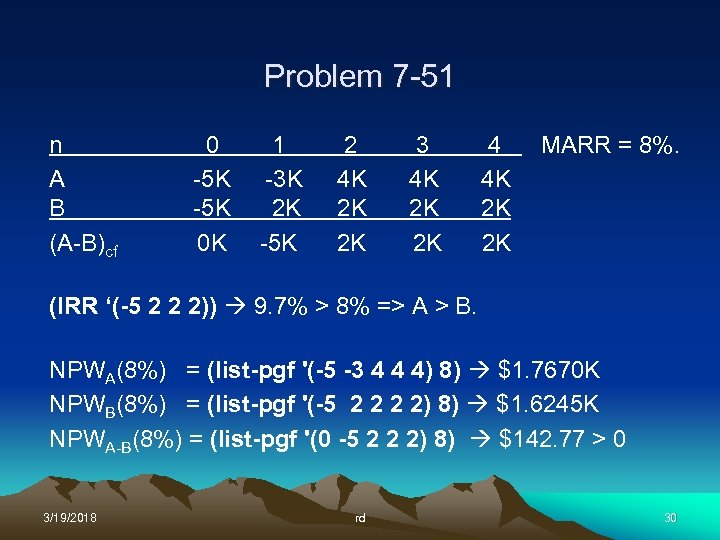Problem 7 -51 n A B (A-B)cf 0 -5 K 0 K 1 -3 K 2 K -5 K 2 4 K 2 K 2 K 3 4 K 2 K 2 K 4 4 K 2 K 2 K MARR = 8%. (IRR ‘(-5 2 2 2)) 9. 7% > 8% => A > B. NPWA(8%) = (list-pgf '(-5 -3 4 4 4) 8) \$1. 7670 K NPWB(8%) = (list-pgf '(-5 2 2) 8) \$1. 6245 K NPWA-B(8%) = (list-pgf '(0 -5 2 2 2) 8) \$142. 77 > 0 3/19/2018 rd 30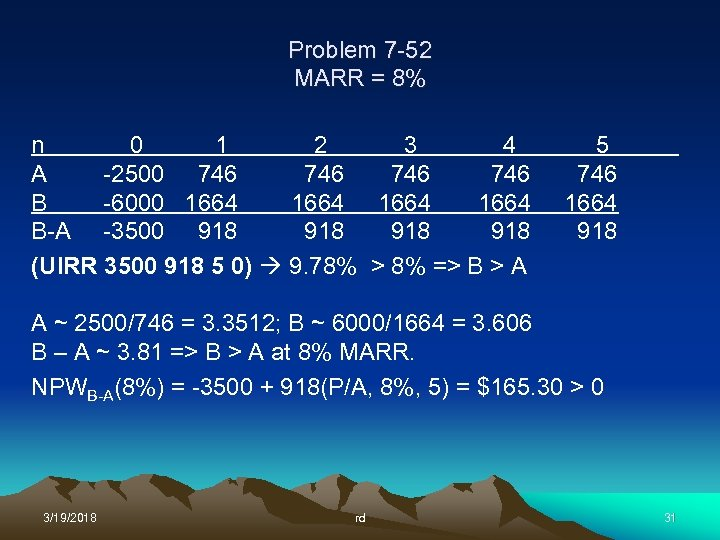Problem 7 -52 MARR = 8% n 0 1 2 3 4 A -2500 746 746 B -6000 1664 B-A -3500 918 918 (UIRR 3500 918 5 0) 9. 78% > 8% => B > A 5 746 1664 918 A ~ 2500/746 = 3. 3512; B ~ 6000/1664 = 3. 606 B – A ~ 3. 81 => B > A at 8% MARR. NPWB-A(8%) = -3500 + 918(P/A, 8%, 5) = \$165. 30 > 0 3/19/2018 rd 31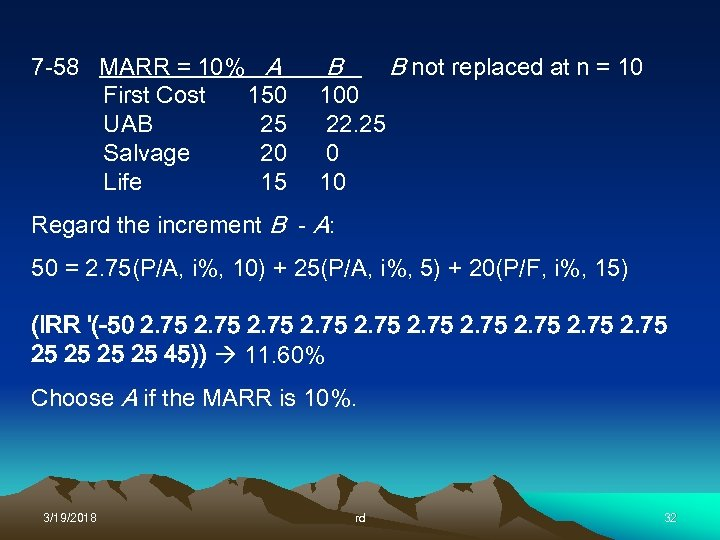7 -58 MARR = 10% A First Cost 150 UAB 25 Salvage 20 Life 15 B 100 22. 25 0 10 B not replaced at n = 10 Regard the increment B - A: 50 = 2. 75(P/A, i%, 10) + 25(P/A, i%, 5) + 20(P/F, i%, 15) (IRR '(-50 2. 75 25 25 45)) 11. 60% Choose A if the MARR is 10%. 3/19/2018 rd 32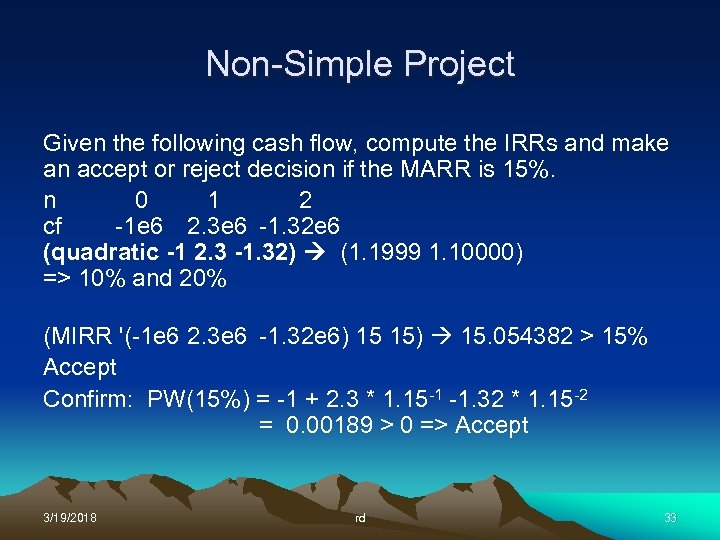Non-Simple Project Given the following cash flow, compute the IRRs and make an accept or reject decision if the MARR is 15%. n 0 1 2 cf -1 e 6 2. 3 e 6 -1. 32 e 6 (quadratic -1 2. 3 -1. 32) (1. 1999 1. 10000) => 10% and 20% (MIRR '(-1 e 6 2. 3 e 6 -1. 32 e 6) 15 15) 15. 054382 > 15% Accept Confirm: PW(15%) = -1 + 2. 3 * 1. 15 -1 -1. 32 * 1. 15 -2 = 0. 00189 > 0 => Accept 3/19/2018 rd 33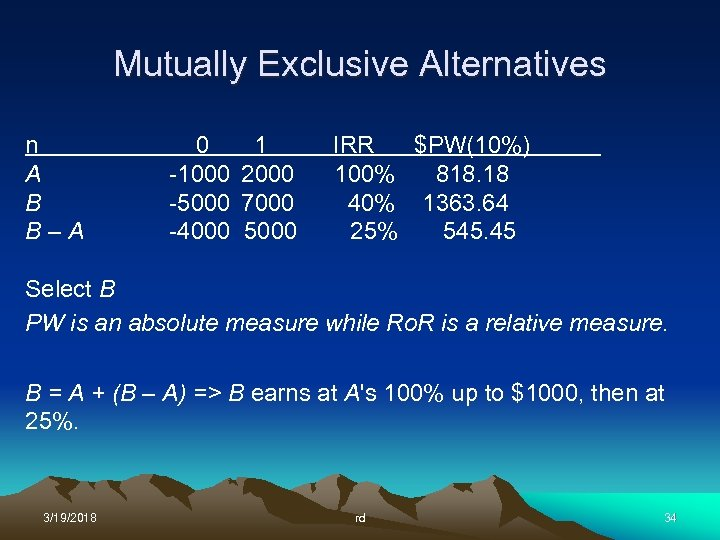Mutually Exclusive Alternatives n A B B–A 0 -1000 -5000 -4000 1 2000 7000 5000 IRR \$PW(10%) 100% 818. 18 40% 1363. 64 25% 545. 45 Select B PW is an absolute measure while Ro. R is a relative measure. B = A + (B – A) => B earns at A's 100% up to \$1000, then at 25%. 3/19/2018 rd 34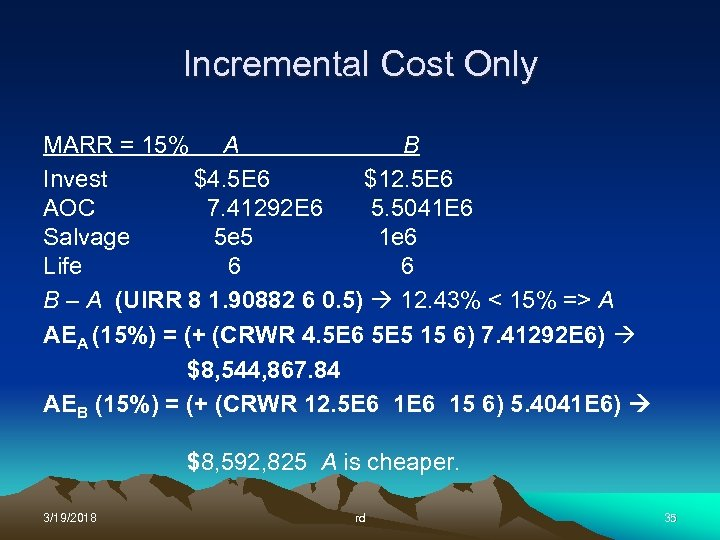Incremental Cost Only MARR = 15% A B Invest \$4. 5 E 6 \$12. 5 E 6 AOC 7. 41292 E 6 5. 5041 E 6 Salvage 5 e 5 1 e 6 Life 6 6 B – A (UIRR 8 1. 90882 6 0. 5) 12. 43% < 15% => A AEA (15%) = (+ (CRWR 4. 5 E 6 5 E 5 15 6) 7. 41292 E 6) \$8, 544, 867. 84 AEB (15%) = (+ (CRWR 12. 5 E 6 15 6) 5. 4041 E 6) \$8, 592, 825 A is cheaper. 3/19/2018 rd 35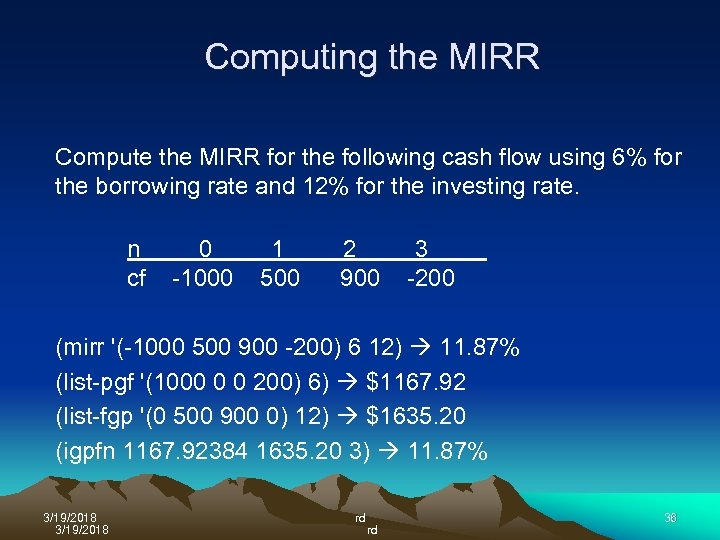Computing the MIRR Compute the MIRR for the following cash flow using 6% for the borrowing rate and 12% for the investing rate. n cf 0 -1000 1 500 2 900 3 -200 (mirr '(-1000 500 900 -200) 6 12) 11. 87% (list-pgf '(1000 0 0 200) 6) \$1167. 92 (list-fgp '(0 500 900 0) 12) \$1635. 20 (igpfn 1167. 92384 1635. 20 3) 11. 87% 3/19/2018 rd rd 36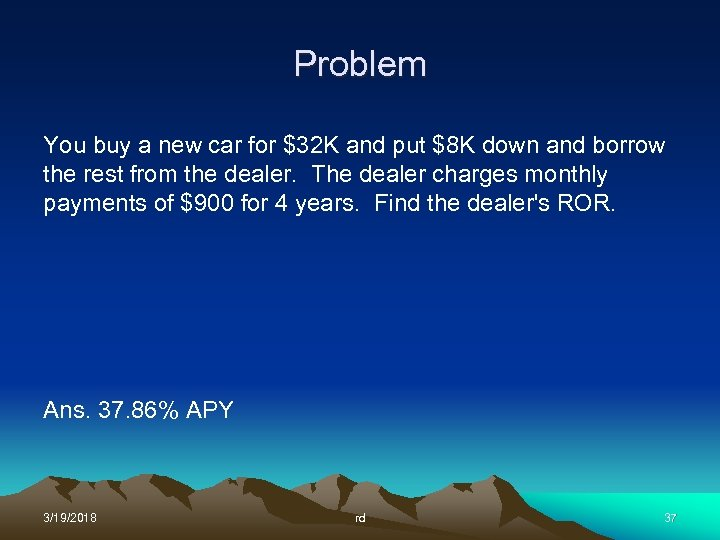Problem You buy a new car for \$32 K and put \$8 K down and borrow the rest from the dealer. The dealer charges monthly payments of \$900 for 4 years. Find the dealer's ROR. Ans. 37. 86% APY 3/19/2018 rd 37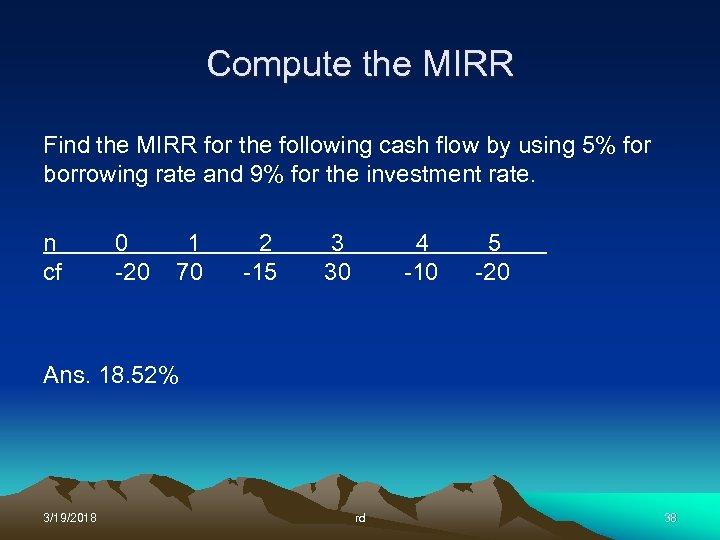Compute the MIRR Find the MIRR for the following cash flow by using 5% for borrowing rate and 9% for the investment rate. n cf 0 -20 1 70 2 -15 3 30 4 -10 5 -20 Ans. 18. 52% 3/19/2018 rd 38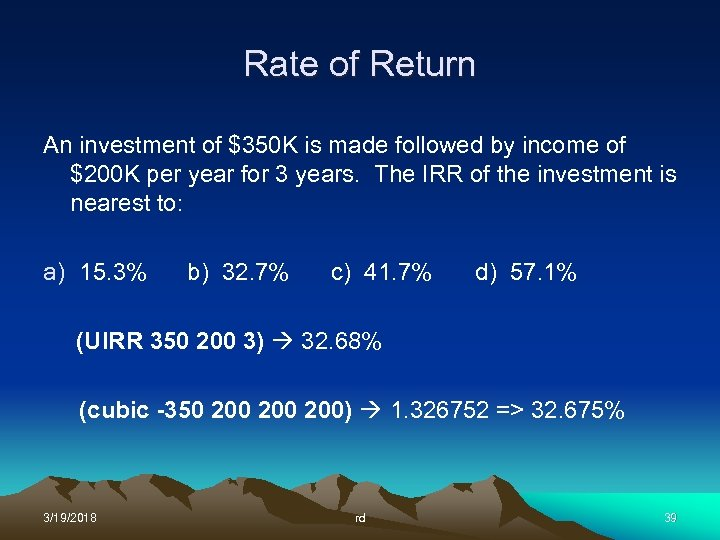Rate of Return An investment of \$350 K is made followed by income of \$200 K per year for 3 years. The IRR of the investment is nearest to: a) 15. 3% b) 32. 7% c) 41. 7% d) 57. 1% (UIRR 350 200 3) 32. 68% (cubic -350 200 200) 1. 326752 => 32. 675% 3/19/2018 rd 39